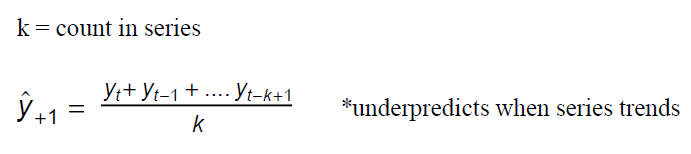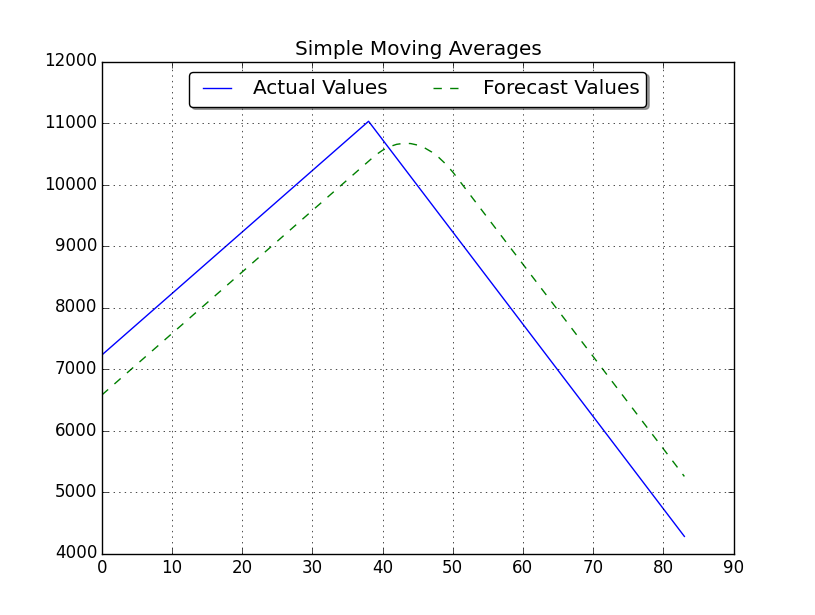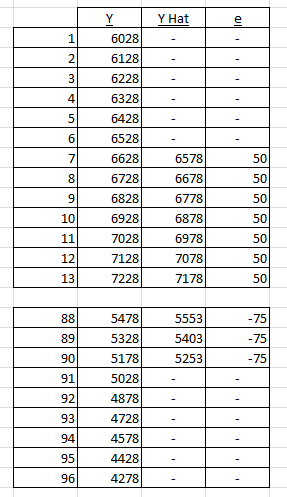# Forecasting and Python Part 1 – Moving Averages

I would like to kick off a series that takes different forecasting methodologies and demonstrates them using Python.  To get the ‘ball rolling’ I want to start with moving averages and ideally end the series on forecasting with ARIMA models (AutoRegressive Integrated Moving Average). My goal is to have this content ‘light’ on theory and mathematics and instead focus on application in code. I do write these late at night, so please feel free to ping me if I have any errors.

I often refer to the predicted Y as Y hat, if you are not aware, in the equation the hat symbol is located over the predicted Y.

### Forecasting with Moving Average

Moving averages should be a a great place to start; every textbook I have starts with moving averages to lay the foundation. The formulas are simple and fun.Equation 1: Moving Averages Equation

The moving averages model computes the mean of each observation in periods k. In my code and results I will be using a 12 period moving average, thus k=12. Y hat (t+1) is the forecast value for next period and Y (t) is the actual value at period t. A period can be hours, days, weeks, months, year, etc. Since the model is the same regardless, I am not going to specify a unit.

```n = len(yvalues)yhat=[]
k = 12

for i in range(0,n):
tmp = 0.0

tmp = math.fsum(yfull[i:i+k])

yhat.append(tmp/k)

```

yvalues is a subset of all the actual Y values brought into the code. Set my ‘k’ (periods) equal to 12 as I am going to calculate a 12 period moving average.

The code iterates over the observations (n), calculates the mean for each 12 period range (k=12) and assigns the calculation to the yhat list. In case you noticed, yfull is my full list of Y values, where yvalues is a subset that has been offset by k.. more on this below in the ‘Offsetting Y Subset List’ section.

### Plot Actual and ForecastForecast 1 :  12 period moving average

```fig = plt.figure(1)
plt.plot(yvalues,label='Actual Values')
plt.plot(yhat,label='Forecast Values',linestyle='--')
plt.title('Simple Moving Averages')
plt.grid(True)plt.legend(loc='upper center',

### Measuring Forecast Errors

I have my forecast, but is it any good? Visually the forecast follows the actual values rather well, but how can I measure the quality of this forecast and then compare it against forecasts derived using different methods. One way to measure a forecast is by measuring the errors (a.k.a. residuals, Y actual – Y hat)

I have chosen to include the following methods for measuring forecast errors in this discussion. Mean Squared Error (MSE) which measures the averages of the squared errors (difference of Y and Y hat). MSE is reported in the same units as the values being estimated (Y), thus it could be said that a forecast is off by ‘10,000 units’. This error could be seen as small if the actual values range in the billions of units.  The error could be seen as large if the actual values only range in the 10’s of thousands. A common problem with the MSE is that it heavily weights large outliers inflating the error measurement.. The Root Mean Squared Error takes the square root of the MSE. RMSE represents the  sample standard deviation of the residuals.

The Mean Absolute Percentage Error (MAPE) is an alternative method that reports out the error as a percentage. Instead of saying the forecast is off by ‘x units’, we could say a forecast is off by 4%.

I frequently use more than one method when comparing forecasts as each has limitations, which sometime can result in spurious measurements by one or two methods.Equation 2 & 3: MSE and MAPEEquation 3: RMSE

```def mse(y,yhat,n):
tmp = 0.0

for i in range(0,n):
tmp += ((y[i]-yhat[i])**2)

return tmp/n

def rmse(y,yhat,n):

return math.sqrt(mse(y,yhat,n))

def mape(y,yhat,n):
tmp = 0.0

for i in range(0,n):
tmp += math.fabs(y[i]-yhat[i])/y[i]

return (tmp/n)

```

MSE: 630,649.39
RMSE: 794.13
MAPE: 10.22%

#### Offsetting Y Subset List

It is interesting that the above forecast (forecast 1) does not ‘fit’ the actual values more effectively – It is a simple data series, I would expect the residuals to be smaller. To calculate the Y hat values for the 12 period moving average model, I use a formula that moves time (t) 12 periods ahead (see equation 1 above). This was how I was originally taught and have examples for in textbooks on my office shelf.

```yfull = [int(y) for sublist in valuesFromFile for y in sublist]
yvalues = yfull[12:len(yfull)]```

This code creates yfull from the loaded data file then creates a subset list starting 12 periods in. Why… because we will use the first 12 period to kick-off our moving average forecast.

The forecast values, however, do not ‘fit’ the actual values as much as I would like. They are either under predicting or over predicting. Another method for moving average forecasting suggest starting the forecast at the midpoint of ‘k’.Forecast 1: Data

### Using the Midpoint of ‘k’Forecast 2: 12 period moving average

##### Forecast 2: Error Measurements

MSE: 7,350.78
RMSE: 85.74
MAPE: 0.86%

Comparing the forecast error measurements of forecast 1 with forecast 2 provides an indication that the second method better suits our data. However… There is a lot of content available on Centered Moving Averages that will give full detail of how to calculate the midpoint values for even/odd periods. I am not that source; I am merely demonstrating how reducing the lag of the averages better aligns our Y hats to the actual and improves the error measures.

```yfull = [int(y) for sublist in valuesFromFile for y in sublist]
yvalues = yfull[6:len(yfull)-6]```

The code is nearly identical, except the subset list (yvalues) is created 6 periods in and stops 6 periods short.  Averaging the data from the midpoint onward reduced the amount of over/under predicting as done in forecast 1.Forecast 2: Data

### When Moving Averages is less Suited

Moving average forecasting begins to really fail when the data series has a cyclical component or seasonality. Below is the same 12 period  moving average Python code against a cyclical data series.Forecast 3:  12 period moving averages

##### Forecast 3: Error Measurements

MSE: 5,386,003,002.91
RMSE: 73,389.39
MAPE: 48.79%

The plot and the calculated error measure both indicate that moving averages is not a good fit for this series. I will use this same series with other forecasting models to demonstrate techniques that do pick-up cycles in the data.

Jonathan

## Your feedback is greatly appreciated.

Click on a star to rate it!

Average rating / 5. Vote count:

No votes so far! Be the first to rate this post.

## We are sorry that this post was not useful for you!

Let us improve this post!

Categories: Forecasting, Python